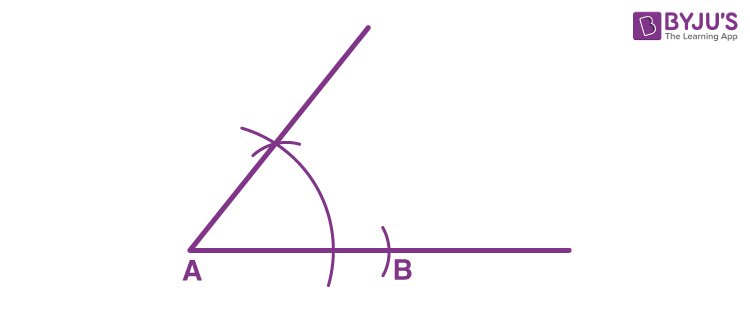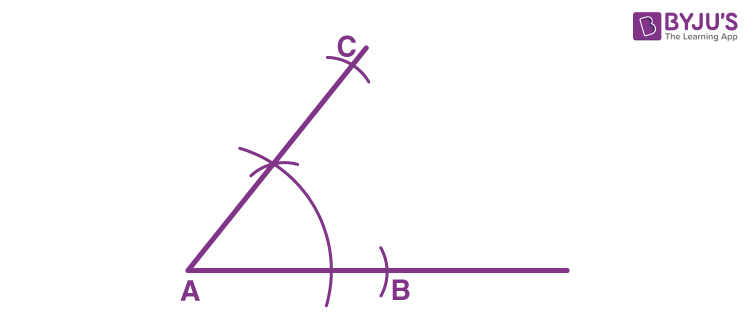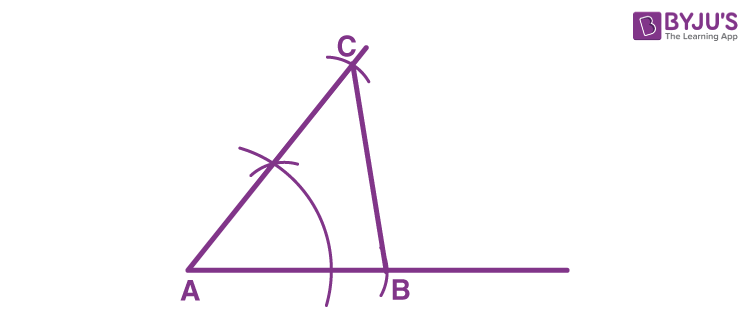Checkout JEE MAINS 2022 Question Paper Analysis : Checkout JEE MAINS 2022 Question Paper Analysis :

# Constructing Triangles, SAS

Constructing SAS triangles includes two known sides of the triangle and one measure of the angle. Students can construct Side-Angle-Side triangle with the help of compass and ruler easily. SAS is one of the properties of similar triangles. Apart from SAS, there are ASA(angle-side-angle), SSS(side-side-side) and AAA(Angle-Angle-Angle). Here we will learn to construct a triangle with side-angle-side similarity.

## SAS Triangle Construction

To satisfy the condition for SAS, two sides and enclosed angle must be given (or known) to us. The construction is not possible when any other angle is provided. It means that it has two line segments and one angle. While constructing the triangle, a triangle should be constructed in a way such that the angle will be included between the two line segments. The order of constructing SAS triangle will be “a copy of a segment followed by the copy of angle and then followed by the copy of the segment”.

Requirements: For the construction of the triangle using SAS criteria we require a Ruler and a Compass.

Let us say, in a triangle ABC, the lengths of the sides are AB = 4 cm, AC = 7 cm and ∠CAB = 60 degrees. The steps for its construction are:

• Step 1: Draw a straight line and mark its left endpoint as A.
• Step 2: Set the compass to a width of 4 cm.
• Step 3: Place the pointer head of the compass at A and cut an arc on the line.
• Step 4: Mark the point as B where the arc crosses the line.• Step 5: Construct a 60-degree angle with line AB at point A.• Step 6: Set the compass to a width of 7 cm.
• Step 7: Place the pointer head of the compass at A and cut an arc on the 60 degree line.
• Step 8: Mark the point as C where the arc crosses the line.• Step 9: Join points B and C using a ruler.Thus, you obtained a triangle ABC of the required measurements.

## Video Lesson on Congruency Rules (SAS and ASA)